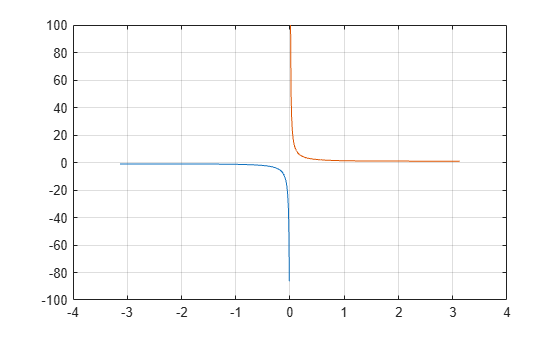# coth

Hyperbolic cotangent

## Syntax

``Y = coth(X)``

## Description

example

````Y = coth(X)` returns the hyperbolic tangent of the elements of `X`. The `coth` function operates element-wise on arrays. The function accepts both real and complex inputs. All angles are in radians.```

## Examples

collapse all

Create a vector and calculate the hyperbolic cotangent of each value.

```X = [0 pi 2*pi 3*pi]; Y = coth(X)```
```Y = 1×4 Inf 1.0037 1.0000 1.0000 ```

Plot the hyperbolic cotangent over the domain $-\pi and $0.

```x1 = -pi+0.01:0.01:-0.01; x2 = 0.01:0.01:pi-0.01; y1 = coth(x1); y2 = coth(x2); plot(x1,y1,x2,y2) grid on```## Input Arguments

collapse all

Input angles in radians, specified as a scalar, vector, matrix, multidimensional array, table, or timetable.

Data Types: `single` | `double` | `table` | `timetable`
Complex Number Support: Yes

collapse all

### Hyperbolic Cotangent

The hyperbolic cotangent of x is equal to the inverse of the hyperbolic tangent

`$\mathrm{coth}\left(x\right)=\frac{1}{\mathrm{tanh}\left(x\right)}=\frac{{e}^{2x}+1}{{e}^{2x}-1}.$`

In terms of the traditional cotangent function with a complex argument, the identity is

`$\mathrm{coth}\left(x\right)=i\mathrm{cot}\left(ix\right)\text{\hspace{0.17em}}.$`

## Version History

Introduced before R2006a

expand all